# Relaxed Mumford-Shah regularization#

In this tutorial we will use a relaxed Mumford-Shah (rMS) functional 1 as regularization, which has the following form:

$\text{rMS}(x) = \min (\alpha\Vert x\Vert_2^2, \kappa).$

Its corresponding proximal operator is given by

$\begin{split}\text{prox}_{\tau \text{rMS}}(x) = \begin{cases} \frac{1}{1+2\tau \alpha}x & \text{ if } & \vert x\vert \leq \sqrt{\frac{\kappa}{\alpha}(1 + 2\tau \alpha)} \\ \kappa & \text{ else } \end{cases}.\end{split}$

rMS is a combination of Tikhonov and TV regularization. Once the rMS hits a certain threshold, the solution will be allowed to jump due to the constant penalty $$\kappa$$, and below this value rMS will be smooth due to Tikhonov regularization. We show three denoising examples: one example that is well-suited for TV regularization and two examples where rMS outperforms TV and Tikhonov regularization, modeled after the experiments in 2.

References

1

Strekalovskiy, E., and D. Cremers, 2014, Real-time minimization of the piecewise smooth Mumford-Shah functional: European Conference on Computer Vision, 127–141

2

Kadu, A., and Kumar, R. and van Leeuwen, Tristan. Full-waveform inversion with Mumford-Shah regularization. SEG International Exposition and Annual Meeting, SEG-2018-2997224

import numpy as np
import matplotlib.pyplot as plt
import pylops
import pyproximal

np.random.seed(1)


We start with a simple model with two jumps that is well-suited for TV regularization

# Create noisy data
nx = 101
idx_jump1 = nx // 3
idx_jump2 = 3 * nx // 4
x = np.zeros(nx)
x[:idx_jump1] = 2
x[idx_jump1:idx_jump2] = 5
n = np.random.normal(0, 0.5, nx)
y = x + n

# Plot the model and the noisy data
fig, axs = plt.subplots(1, 1, figsize=(6, 5))
axs.plot(x, label='True model')
axs.plot(y, label='Noisy model')
axs.legend()
plt.tight_layout()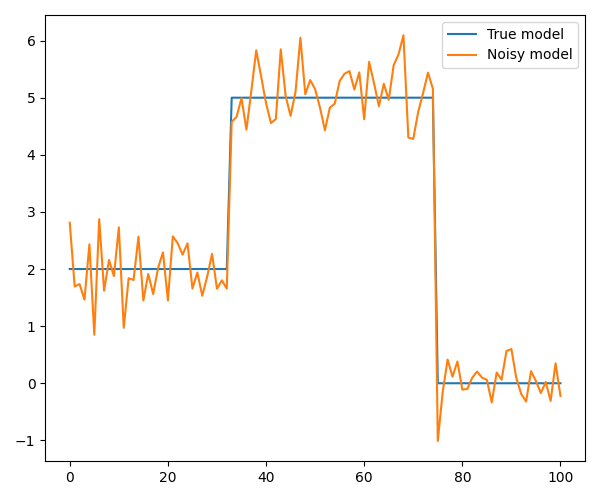For both rMS and TV regularizations we use the Linearized ADMM, whilst for Tikhonov regularization we use LSQR

# Define functionals
l2 = pyproximal.proximal.L2(b=y)
l1 = pyproximal.proximal.L1(sigma=5.)
Dop = pylops.FirstDerivative(nx, edge=True, kind='backward')

# TV
L = np.real((Dop.H * Dop).eigs(neigs=1, which='LM'))
tau = 1.
mu = 0.99 * tau / L
xTV, _ = pyproximal.optimization.primal.LinearizedADMM(l2, l1, Dop, tau=tau, mu=mu,
x0=np.zeros_like(x), niter=200)

# rMS
sigma = 1e5
kappa = 1e0
ms_relaxed = pyproximal.proximal.RelaxedMumfordShah(sigma=sigma, kappa=kappa)
tau = 1.
mu = tau / L
xrMS, _ = pyproximal.optimization.primal.LinearizedADMM(l2, ms_relaxed, Dop, tau=tau, mu=mu,
x0=np.zeros_like(x), niter=200)

# Tikhonov
xTikhonov = pylops.optimization.leastsquares.regularized_inversion(Op=pylops.Identity(nx),
Regs=[Dop, ], y=y,
epsRs=[6e0, ])

# Plot the results
fig, axs = plt.subplots(1, 1, figsize=(6, 5))
axs.plot(x, label='True', linewidth=4, color='k')
axs.plot(y, '--', label='Noisy', linewidth=2, color='y')
axs.plot(xTV, label='TV')
axs.plot(xrMS, label='rMS')
axs.plot(xTikhonov, label='Tikhonov')
axs.legend()
plt.tight_layout()Next, we consider an example where we replace the first jump with a slope. As we will see, TV can not deal with this type of structure since a linear increase will greatly increase the TV norm, and instead TV will make a staircase. rMS, on the other hand, can reconstruct the model with high accuracy.

nx = 101
idx_jump1 = nx // 3
idx_jump2 = 3 * nx // 4
x = np.zeros(nx)
x[:idx_jump1] = 2
x[idx_jump1:idx_jump2] = np.linspace(2, 4, idx_jump2 - idx_jump1)
n = np.random.normal(0, 0.25, nx)
y = x + n

# Plot the model and the noisy data
fig, axs = plt.subplots(1, 1, figsize=(6, 5))
axs.plot(x, label='True model')
axs.plot(y, label='Noisy model')
axs.legend()
plt.tight_layout()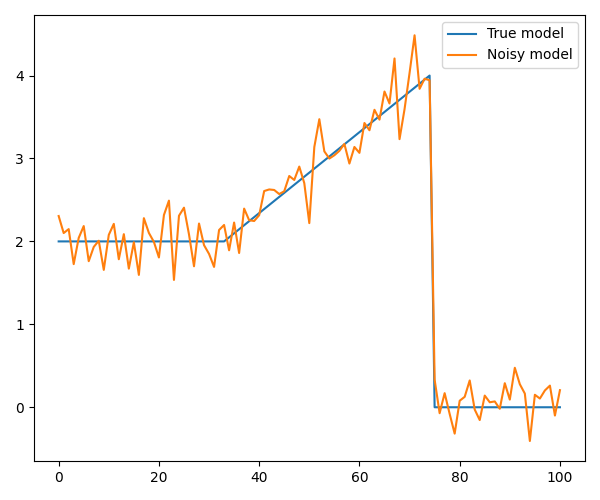# Define functionals
l2 = pyproximal.proximal.L2(b=y)
l1 = pyproximal.proximal.L1(sigma=1.)
Dop = pylops.FirstDerivative(nx, edge=True, kind='backward')

# TV
L = np.real((Dop.H * Dop).eigs(neigs=1, which='LM'))
tau = 1.
mu = 0.99 * tau / L
xTV, _ = pyproximal.optimization.primal.LinearizedADMM(l2, l1, Dop, tau=tau, mu=mu,
x0=np.zeros_like(x), niter=200)

# rMS
sigma = 1e1
kappa = 1e0
ms_relaxed = pyproximal.proximal.RelaxedMumfordShah(sigma=sigma, kappa=kappa)
tau = 1.
mu = tau / L
xrMS, _ = pyproximal.optimization.primal.LinearizedADMM(l2, ms_relaxed, Dop, tau=tau, mu=mu,
x0=np.zeros_like(x), niter=200)

# Tikhonov
Op = pylops.Identity(nx)
Regs = [Dop, ]
epsR = [3e0, ]

xTikhonov = pylops.optimization.leastsquares.regularized_inversion(Op=Op, Regs=Regs, y=y, epsRs=epsR)

# Plot the results
fig, axs = plt.subplots(1, 1, figsize=(6, 5))
axs.plot(x, label='True', linewidth=4, color='k')
axs.plot(y, '--', label='Noisy', linewidth=2, color='y')
axs.plot(xTV, label='TV')
axs.plot(xrMS, label='rMS')
axs.plot(xTikhonov, label='Tikhonov')
axs.legend()
plt.tight_layout()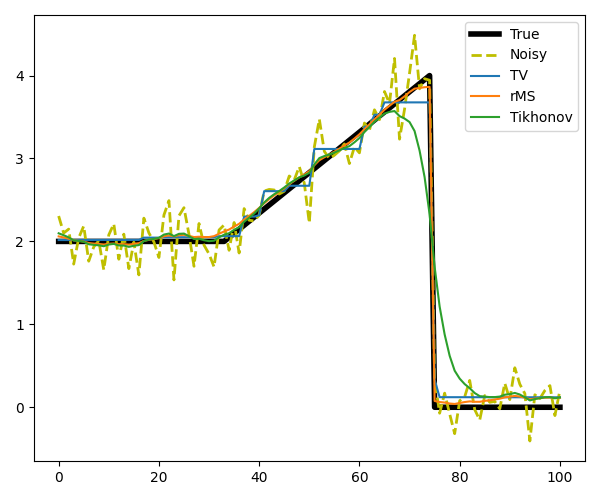Finally, we take a trace from a section of the Marmousi model. This trace shows rather smooth behavior with a few jumps, which makes it perfectly suited for rMS. TV on the other hand will artificially create a staircasing effect.

# Get a trace from the model and add some noise
nz = len(m_trace)
m_trace_noisy = m_trace + np.random.normal(0, 0.1, nz)

# Plot the model and the noisy data
fig, ax = plt.subplots(1, 1, figsize=(12, 5))
ax.plot(m_trace, linewidth=2, label='True')
ax.plot(m_trace_noisy, label='Noisy')
ax.set_title('Trace and noisy trace')
ax.axis('tight')
ax.legend()
plt.tight_layout()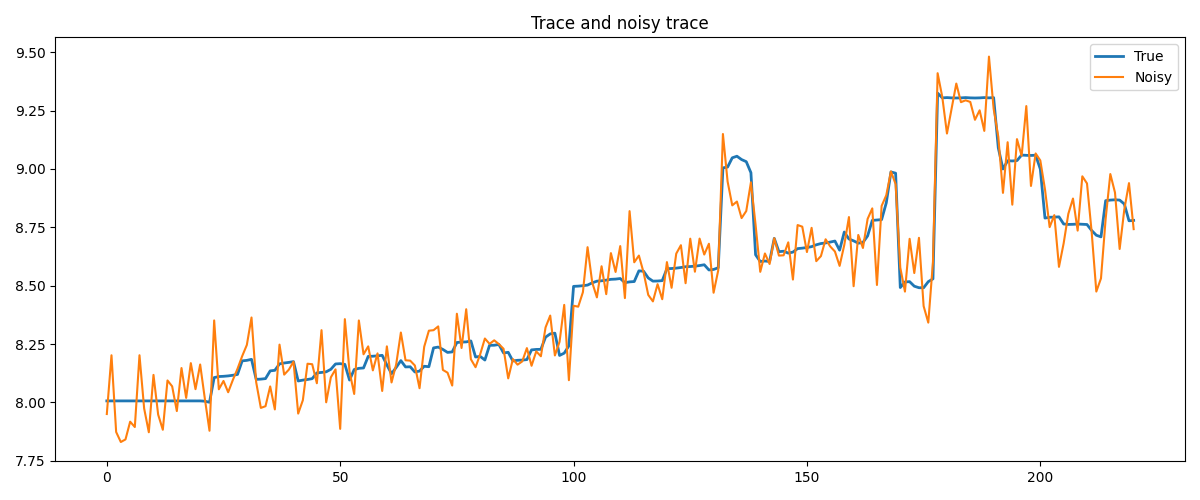# Define functionals
l2 = pyproximal.proximal.L2(b=m_trace_noisy)
l1 = pyproximal.proximal.L1(sigma=5e-1)
Dop = pylops.FirstDerivative(nz, edge=True, kind='backward')

# TV
L = np.real((Dop.H * Dop).eigs(neigs=1, which='LM'))
tau = 1.
mu = 0.99 * tau / L
xTV, _ = pyproximal.optimization.primal.LinearizedADMM(l2, l1, Dop, tau=tau, mu=mu,
x0=np.zeros_like(m_trace), niter=200)

# rMS
sigma = 5e0
kappa = 1e-1
ms_relaxed = pyproximal.proximal.RelaxedMumfordShah(sigma=sigma, kappa=kappa)

tau = 1.
mu = tau / L
xrMS, _ = pyproximal.optimization.primal.LinearizedADMM(l2, ms_relaxed, Dop, tau=tau, mu=mu,
x0=np.zeros_like(m_trace), niter=200)

# Tikhonov
Op = pylops.Identity(nz)
Regs = [Dop, ]
epsR = [3e0, ]

xTikhonov = pylops.optimization.leastsquares.regularized_inversion(Op=Op, Regs=Regs,
y=m_trace_noisy,
epsRs=epsR)

# Plot the results
fig, axs = plt.subplots(1, 1, figsize=(12, 5))
axs.plot(m_trace, label='True', linewidth=4, color='k')
axs.plot(m_trace_noisy, '--', label='Noisy', linewidth=2, color='y')
axs.plot(xTV, label='TV')
axs.plot(xrMS, label='rMS')
axs.plot(xTikhonov, label='Tikhonov')
axs.legend()
plt.tight_layout()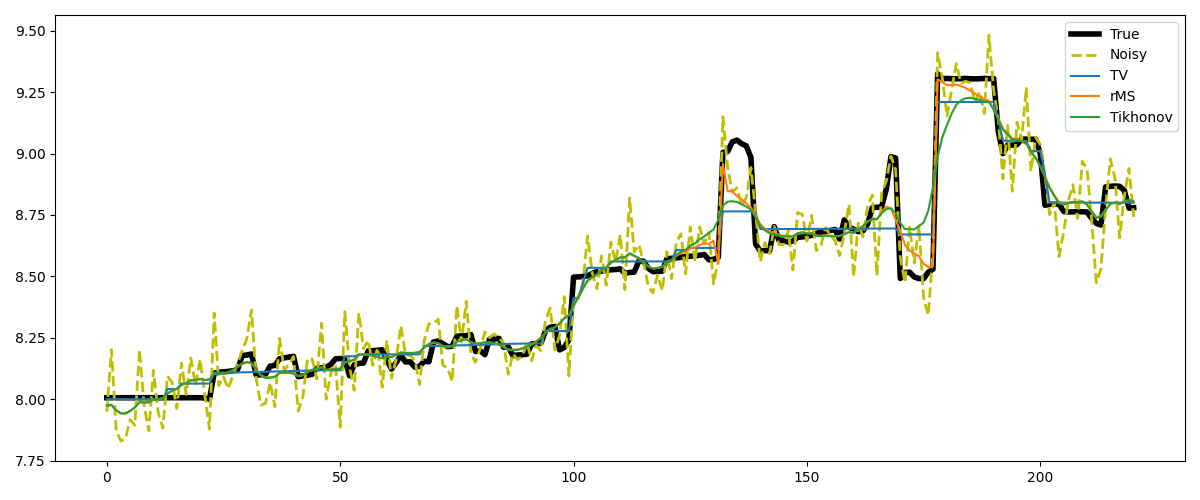Total running time of the script: (0 minutes 1.491 seconds)

Gallery generated by Sphinx-Gallery